The usual way to compute time-variance is given by equation (2). I put here again for your convenient: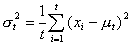(2)

Changing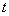to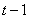makes equation (2) becomes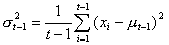or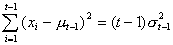(a)

Putting out the last term from equation (2) gives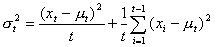Since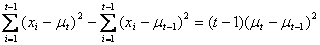then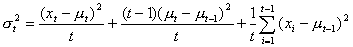(b) ( why? )

Equation (3) can be rearranged into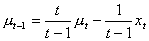(c)

The second term of the right hand side of equation (b) can be manipulated as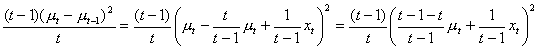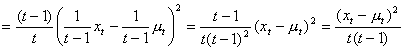Put back the second term into equation (b) and replace the third term with equation (a) becomes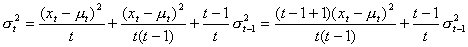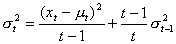(proved)

Preferable reference for this tutorial is

Teknomo, Kardi. (2006) Recursive Average and Variance.
http://people.revoledu.com/kardi/tutorial/RecursiveStatistic/index.html